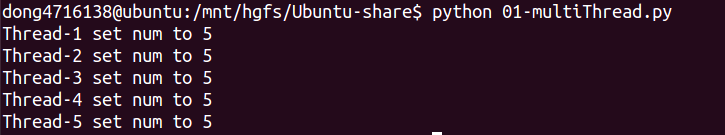# 互斥锁

## 1. 线程不安全举例

``````    #coding=utf-8
import time

num = 0

def run(self):
global num

num = num+1
time.sleep(0.5) #用来模拟适当的数据处理
msg = self.name+' set num to '+str(num)
print msg

def test():
for i in range(5):
t.start()

if __name__ == '__main__':
test()
``````## 2. 互斥锁``````#创建锁
#锁定
mutex.acquire([blocking])
#释放
mutex.release()
``````

• 如果设定blocking为True，则当前线程会堵塞，直到获取到这个锁为止（如果没有指定，那么默认为True）
• 如果设定blocking为False，则当前线程不会堵塞

``````    #coding=utf-8
import time

num = 0

def run(self):
global num
mutexFlag = mutex.acquire(True) #True表示堵塞
print('---线程(%s)的锁状态为%d---'%(self.name,mutexFlag))
if mutexFlag:#判断上锁是否成功
num = num+1
time.sleep(1)
msg = self.name+' set num to '+str(num)
print msg
mutex.release()

def test():
for i in range(5):
t.start()

if __name__ == '__main__':
test()
````````````    #coding=utf-8
import time

num = 0

def run(self):
global num
mutexFlag = mutex.acquire(False) #False表示非堵塞，即不管本次调用能够成功上锁，都不会卡在这,而是继续执行下面的代码
print('---线程(%s)的锁状态为%d---'%(self.name,mutexFlag))
if mutexFlag:#判断上锁是否成功
num = num+1
time.sleep(1)
msg = self.name+' set num to '+str(num)
print msg
mutex.release()

def test():
for i in range(5):
t.start()

if __name__ == '__main__':
test()
``````## 4. 总结

• 确保了某段关键代码只能由一个线程从头到尾完整地执行

• 阻止了多线程并发执行，包含锁的某段代码实际上只能以单线程模式执行，效率就大大地下降了
• 由于可以存在多个锁，不同的线程持有不同的锁，并试图获取对方持有的锁时，可能会造成死锁